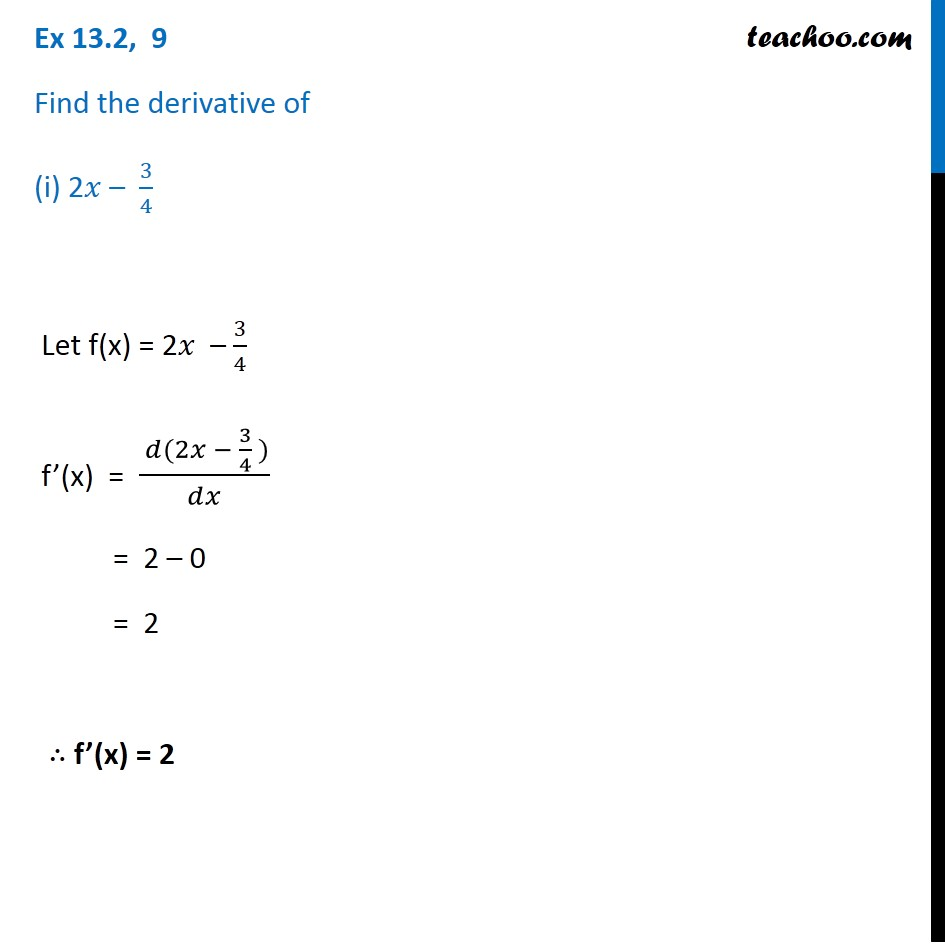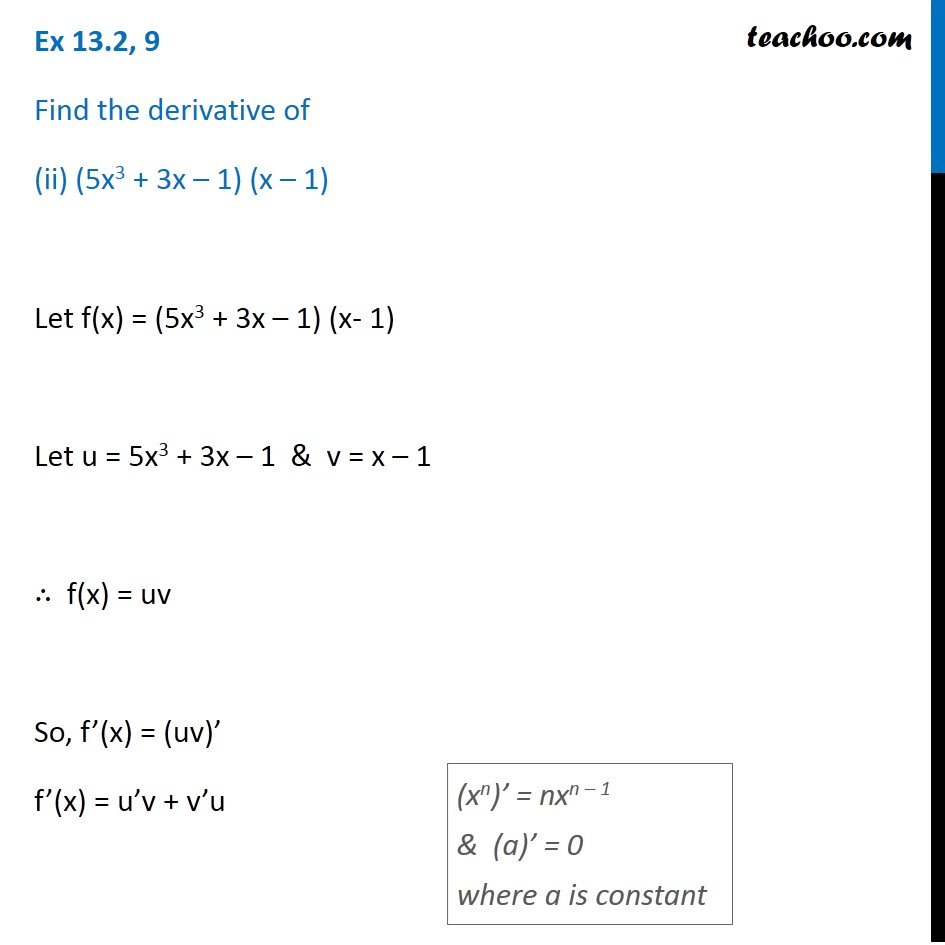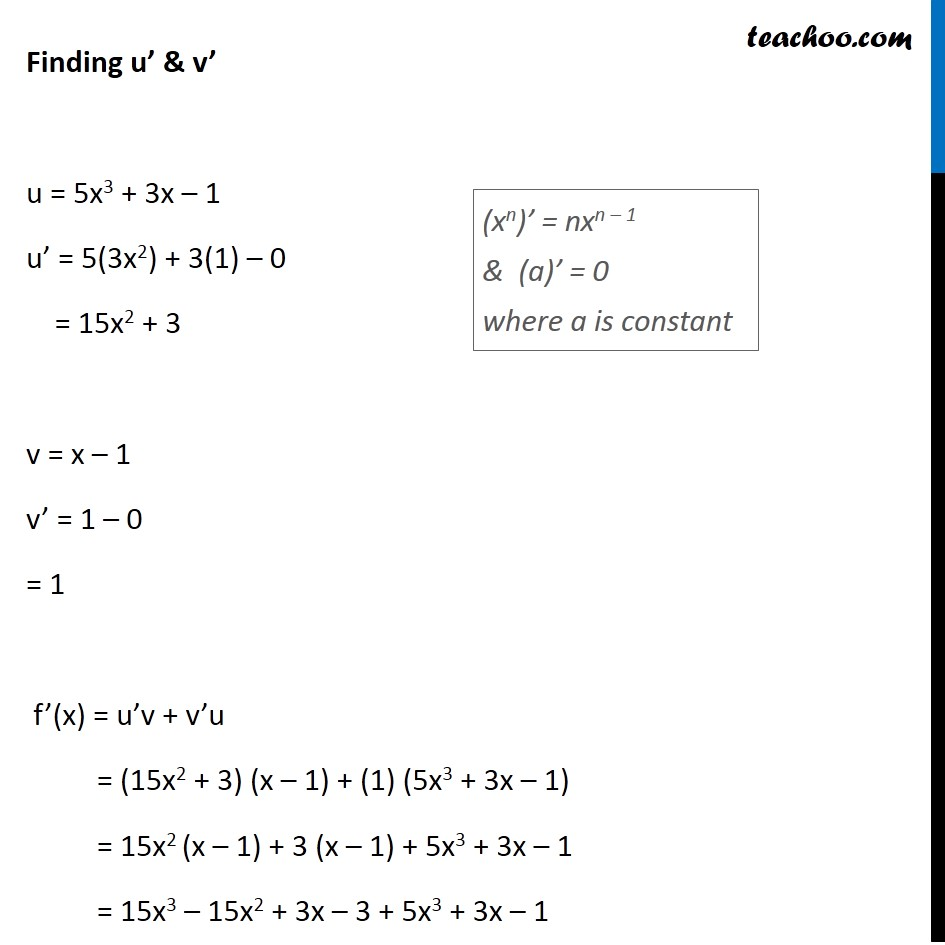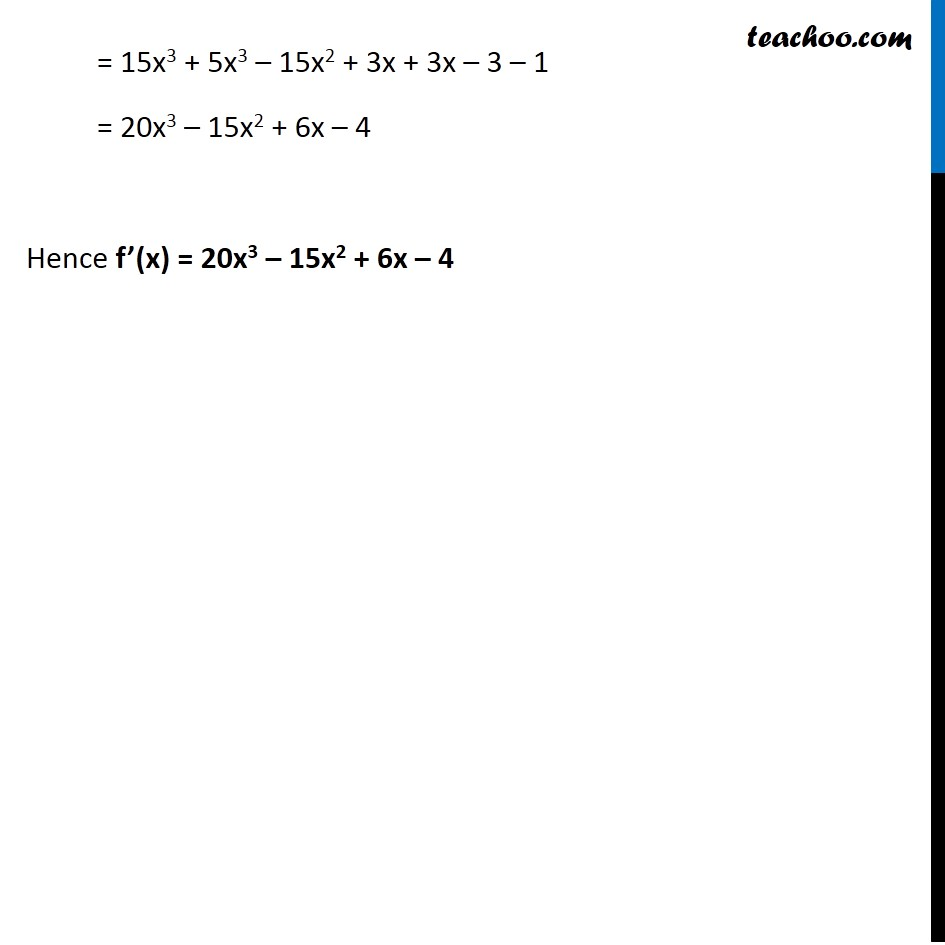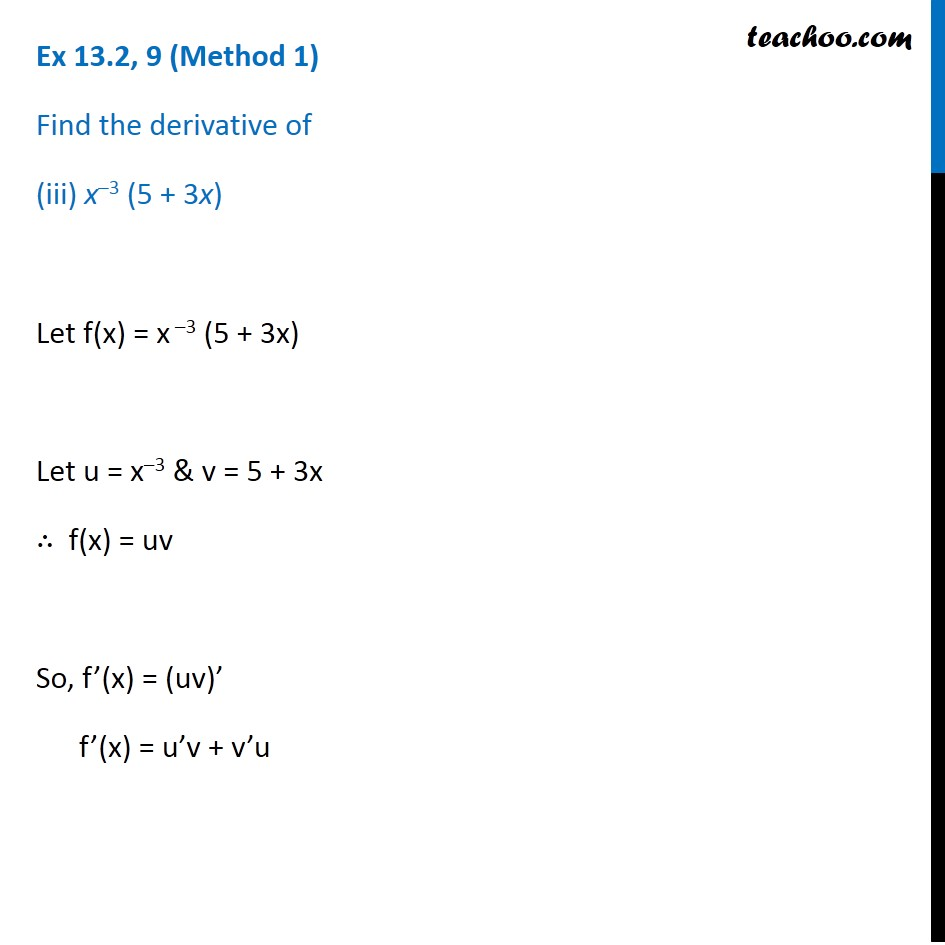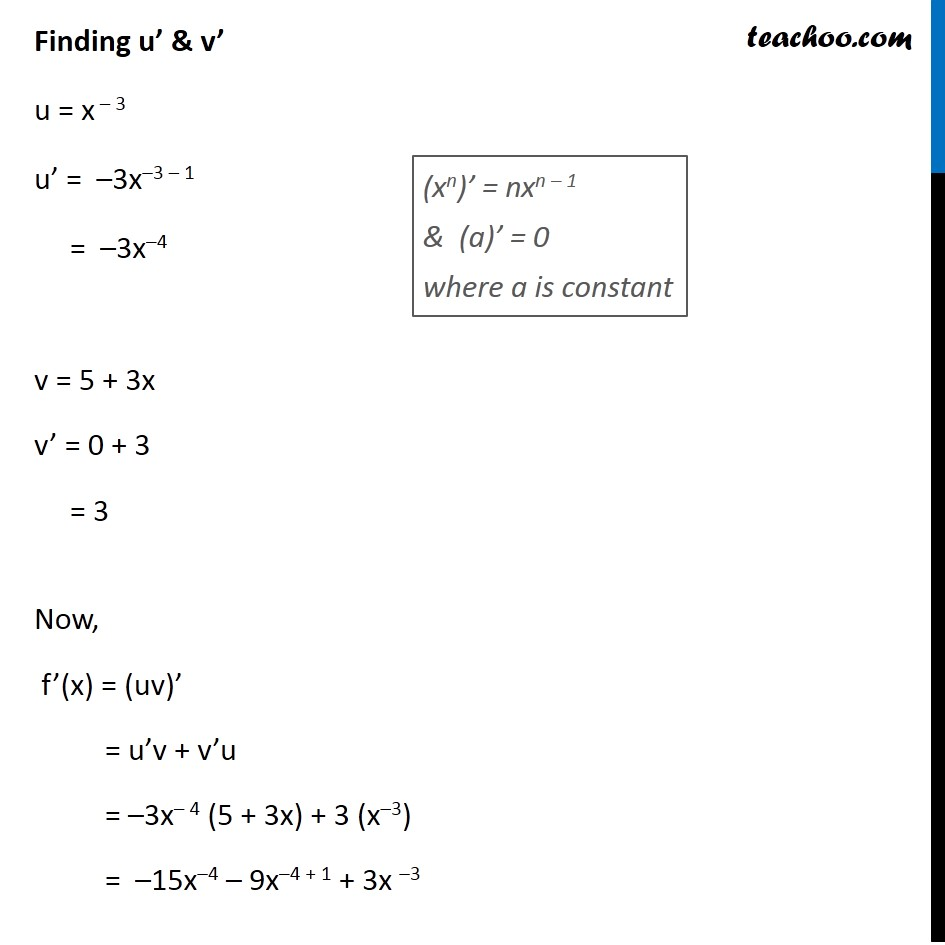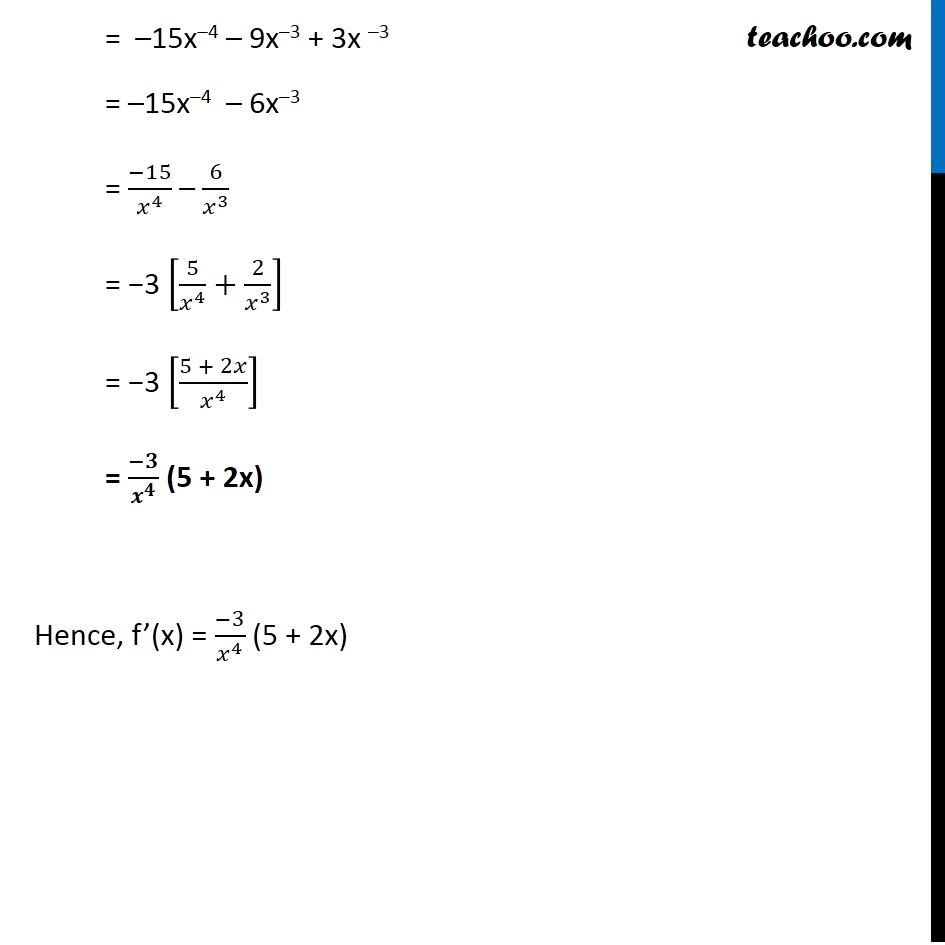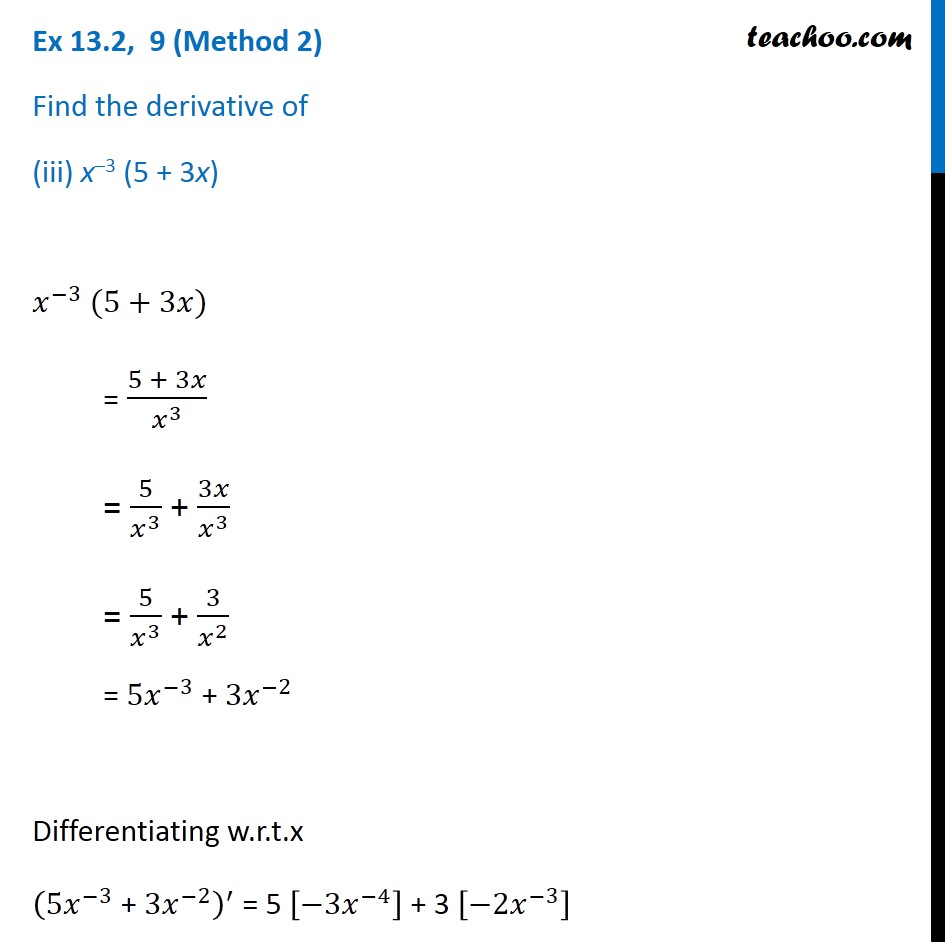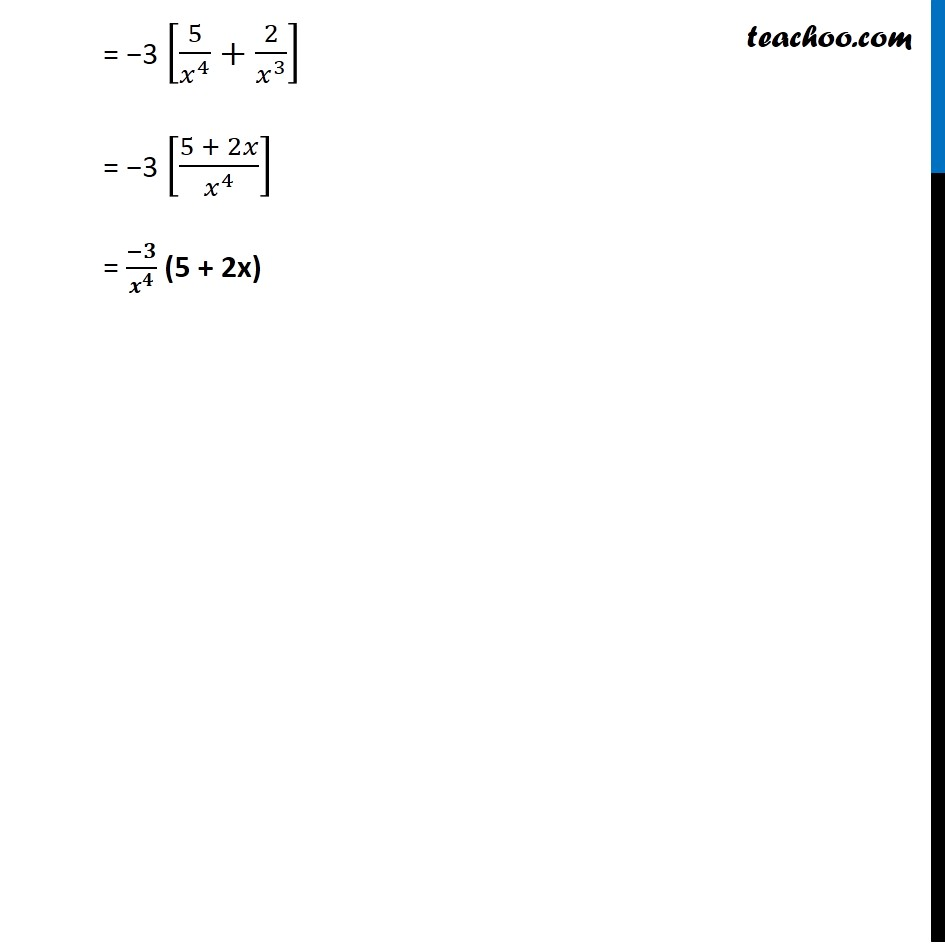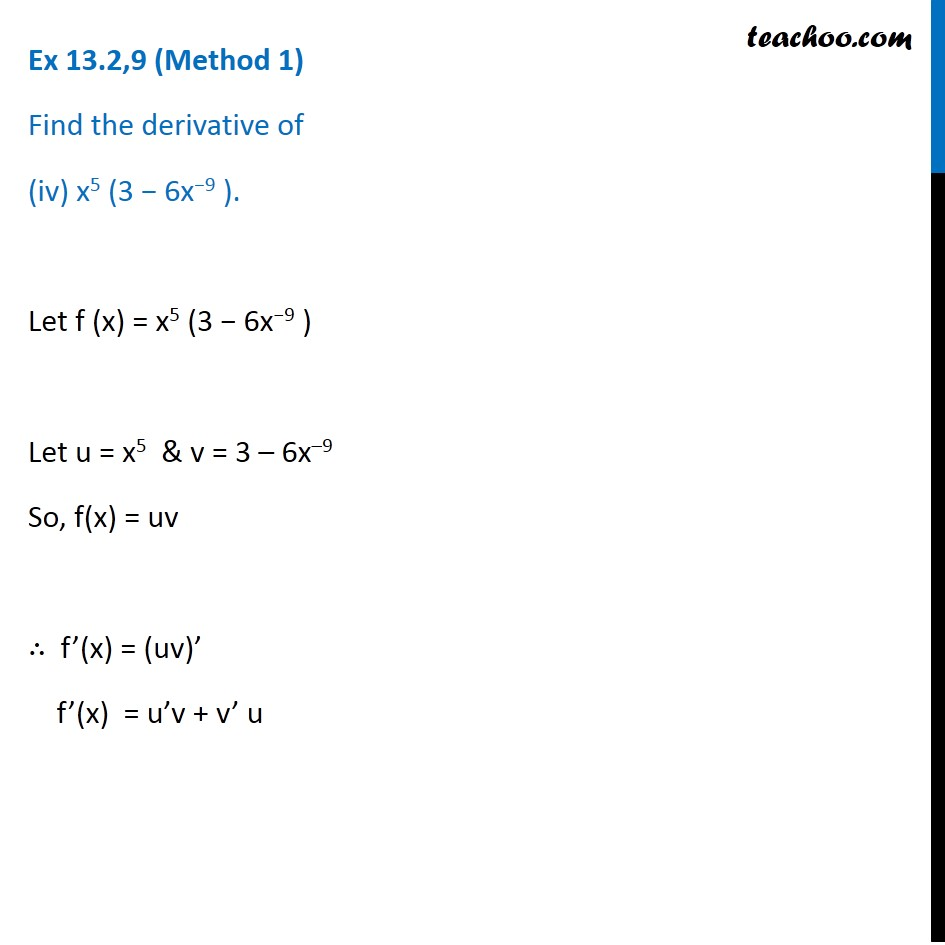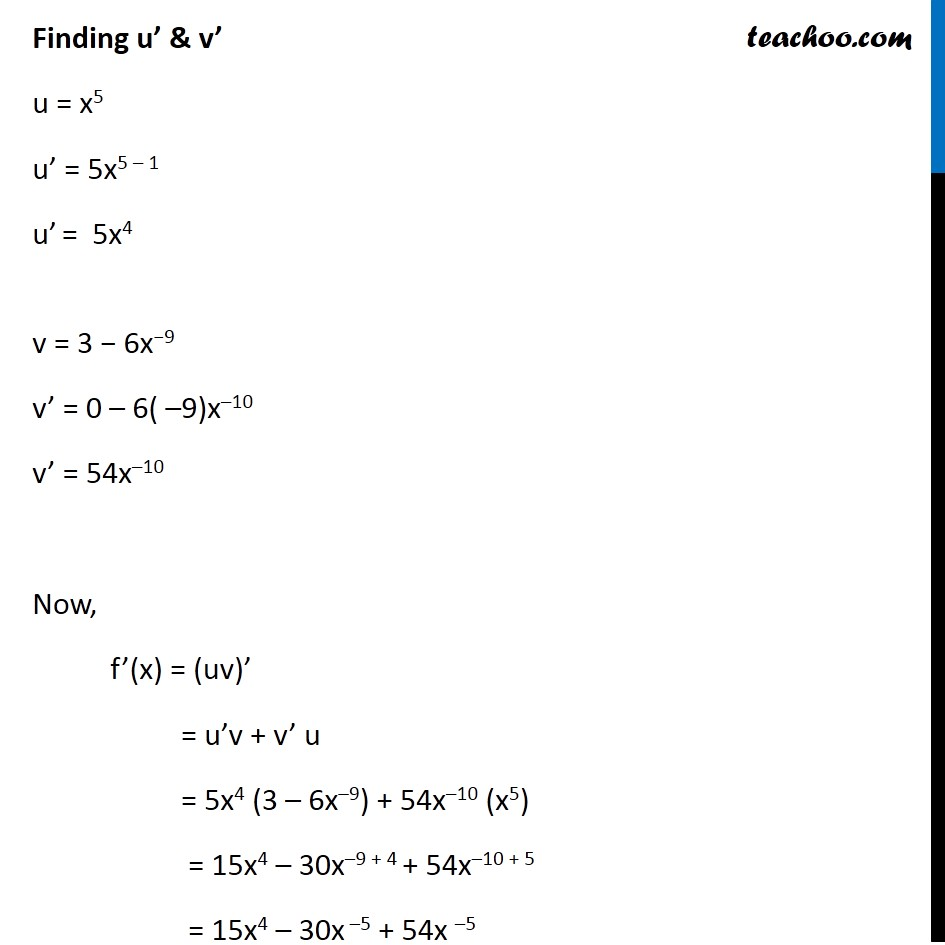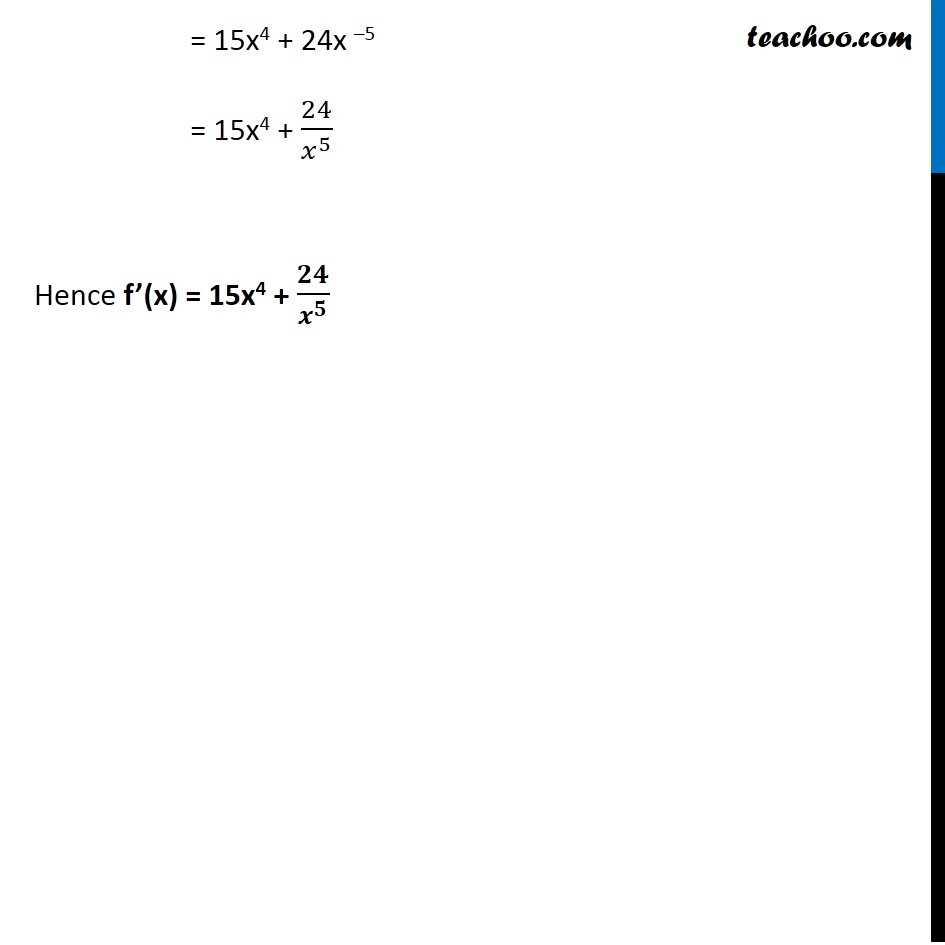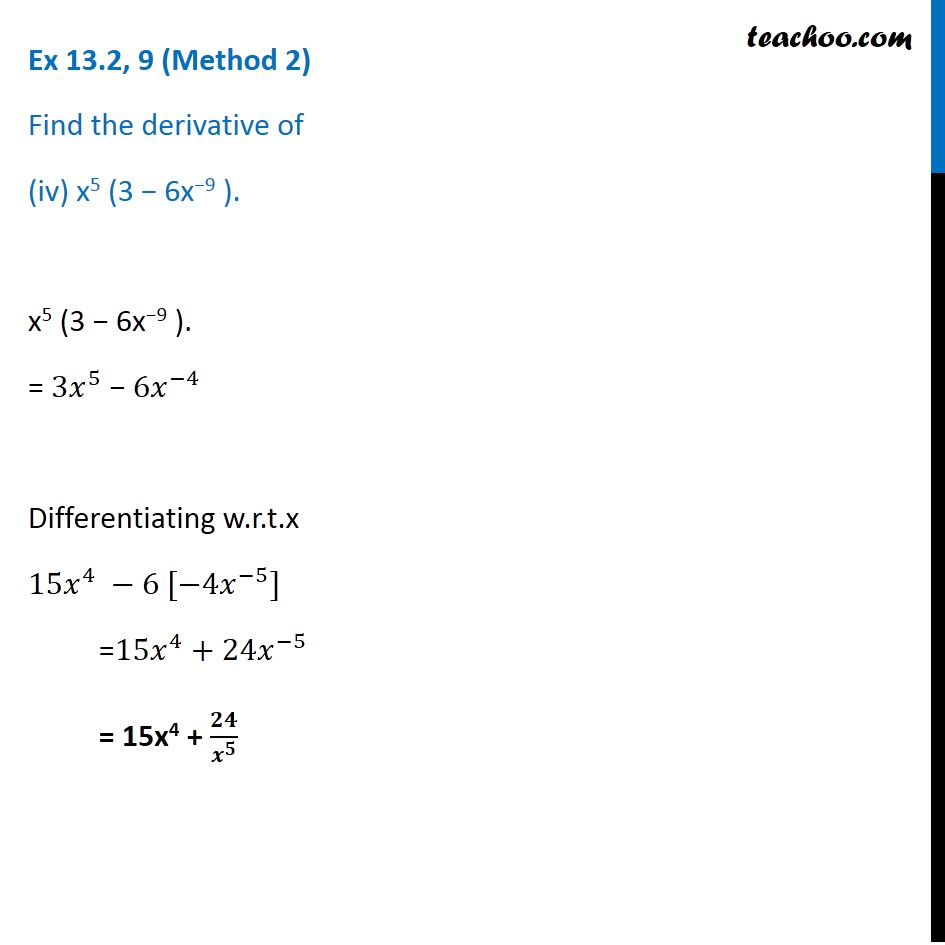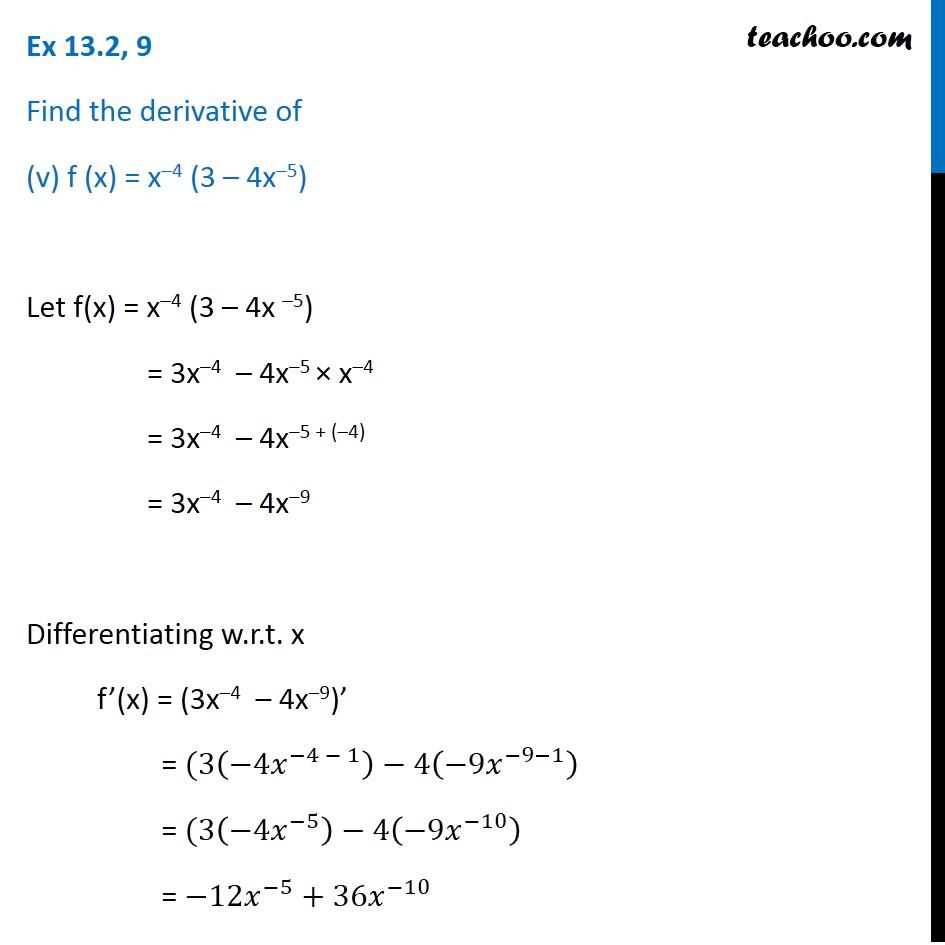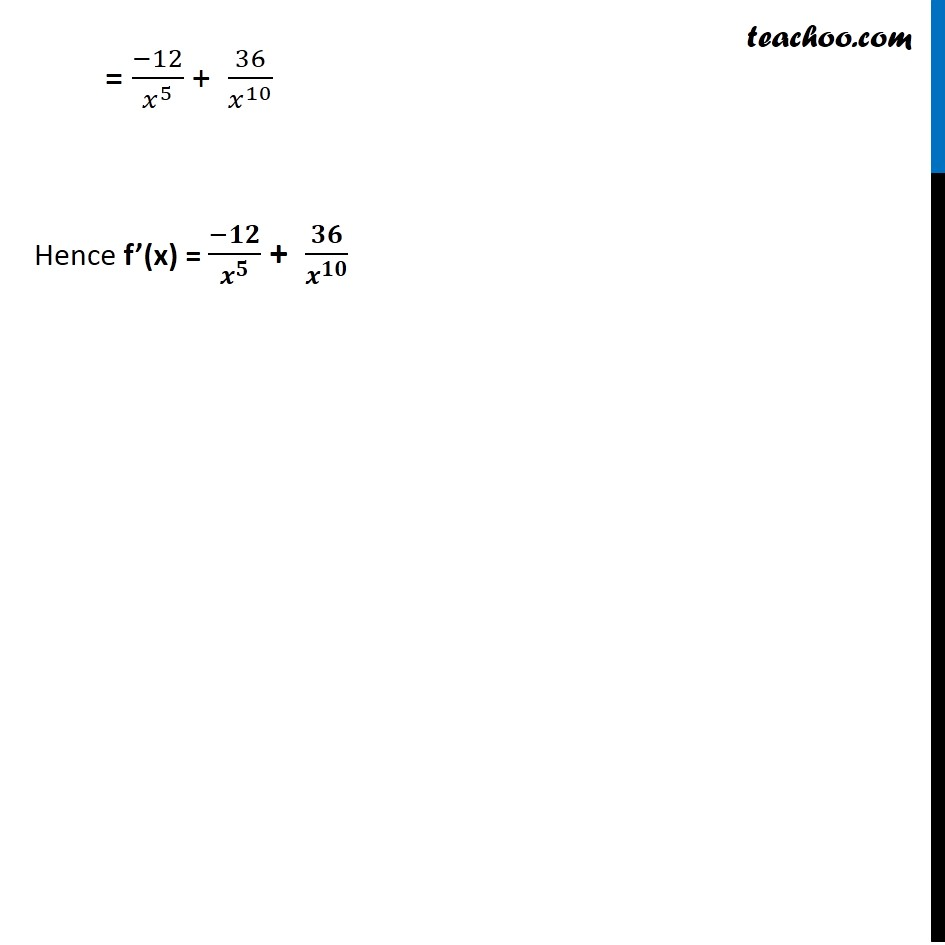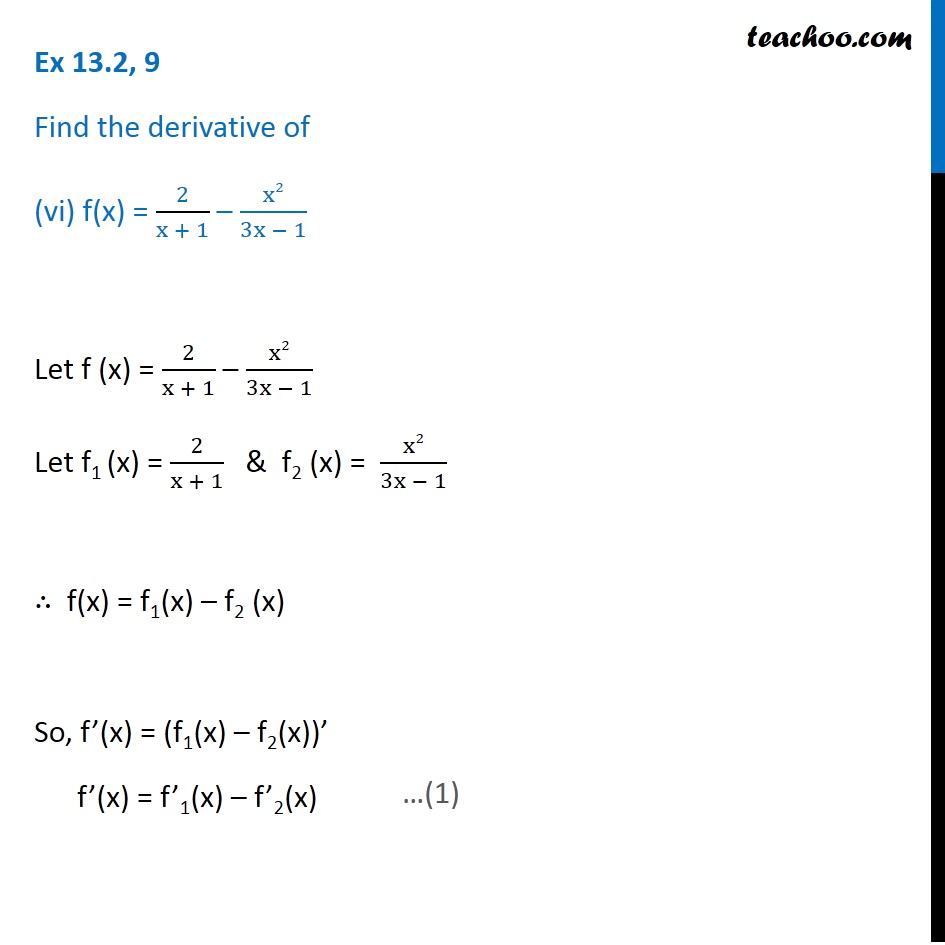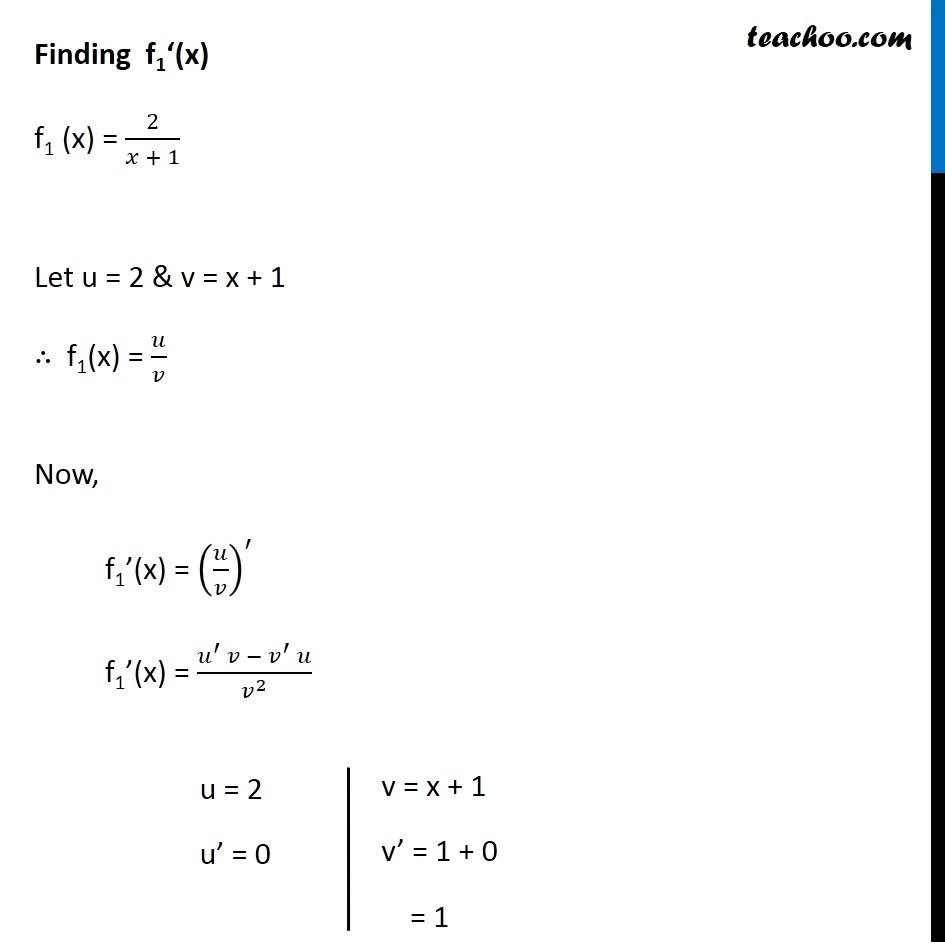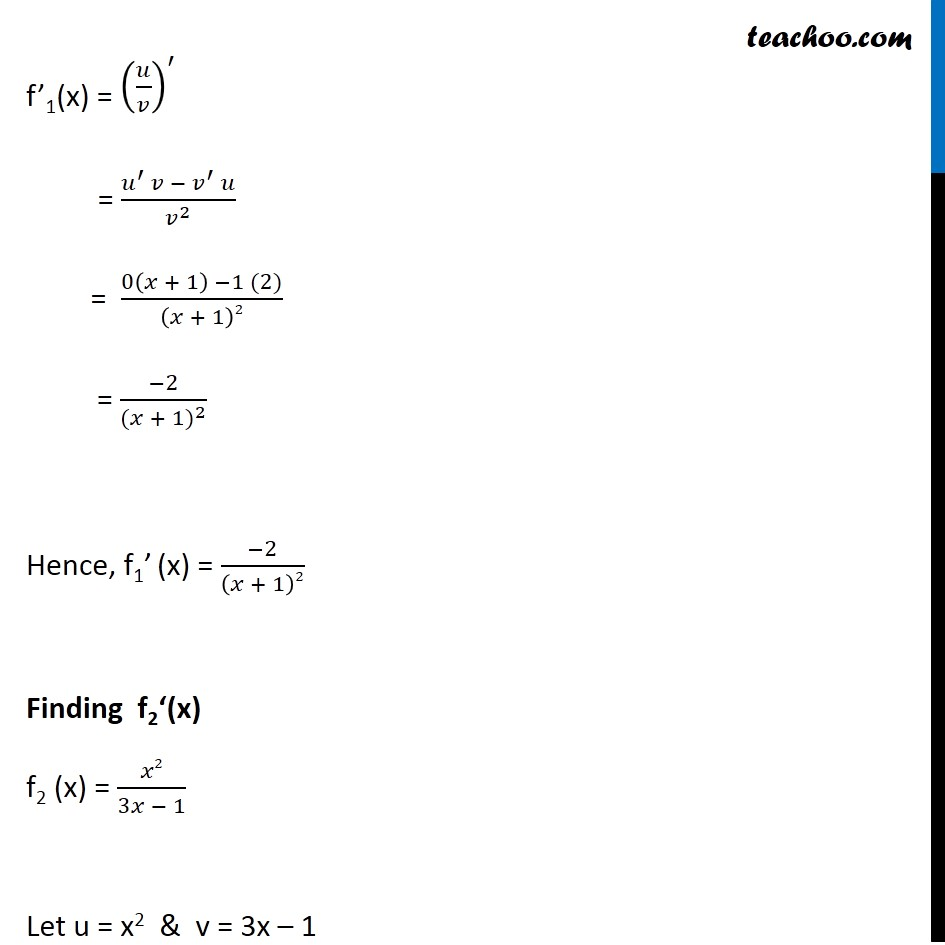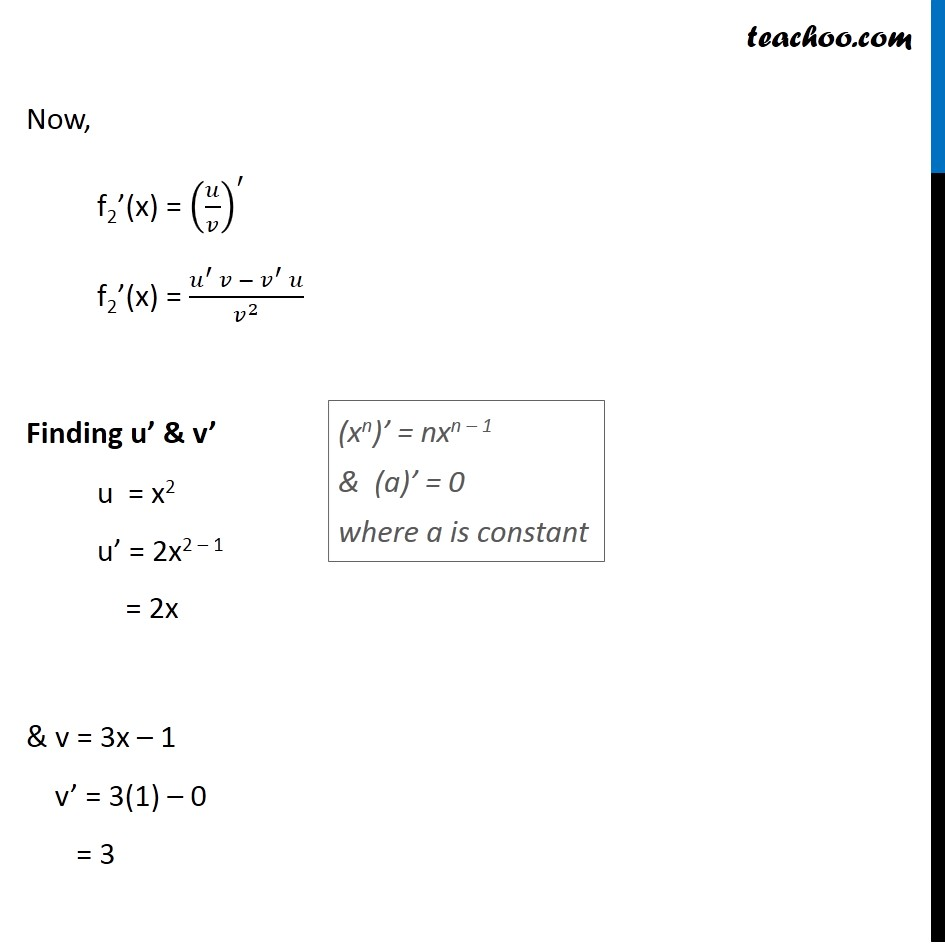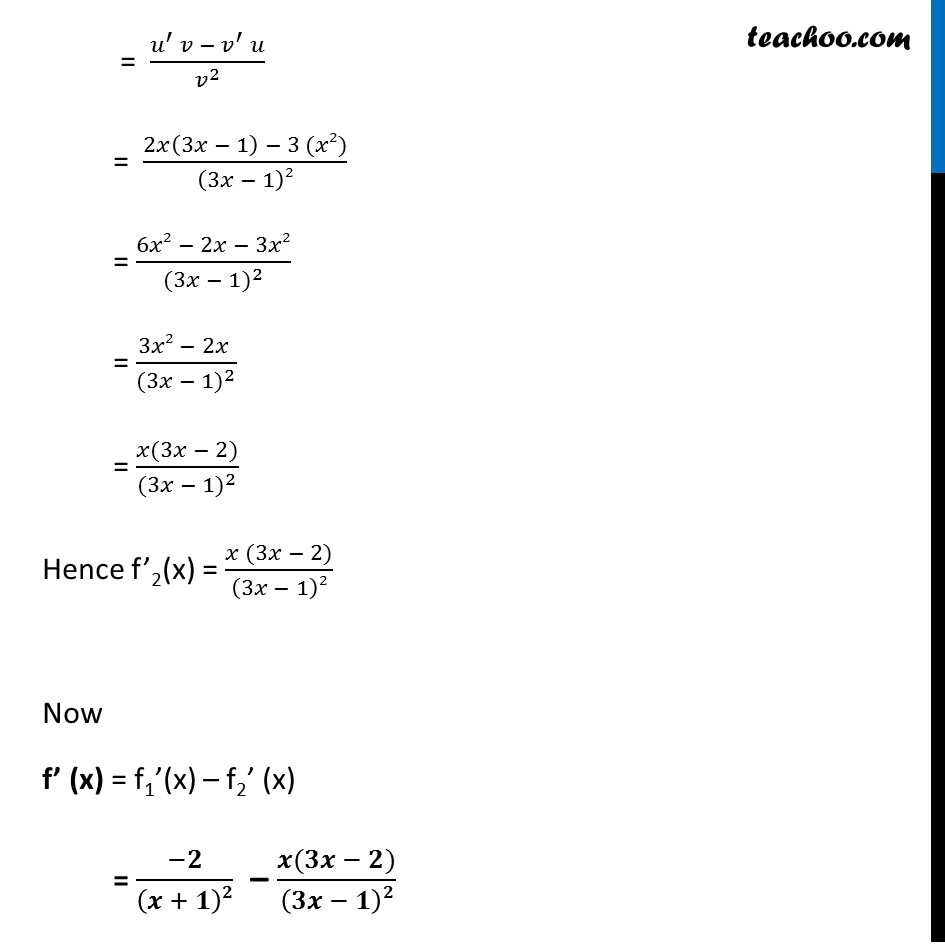1. Chapter 13 Class 11 Limits and Derivatives (Term 1 and Term 2)
2. Serial order wise
3. Ex 13.2 (Term 2)

Transcript

Ex 13.2, 9 Find the derivative of (i) 2𝑥 – 3/4 Let f(x) = 2𝑥 – 3/4 f’(x) = ( 𝑑(2𝑥 − 3/4 ))/𝑑𝑥 = 2 – 0 = 2 ∴ f’(x) = 2 Ex 13.2, 9 Find the derivative of (ii) (5x3 + 3x – 1) (x – 1) Let f(x) = (5x3 + 3x – 1) (x- 1) Let u = 5x3 + 3x – 1 & v = x – 1 ∴ f(x) = uv So, f’(x) = (uv)’ f’(x) = u’v + v’u (xn)’ = nxn – 1 & (a)’ = 0 where a is constant Finding u’ & v’ u = 5x3 + 3x – 1 u’ = 5(3x2) + 3(1) – 0 = 15x2 + 3 v = x – 1 v’ = 1 – 0 = 1 f’(x) = u’v + v’u = (15x2 + 3) (x – 1) + (1) (5x3 + 3x – 1) = 15x2 (x – 1) + 3 (x – 1) + 5x3 + 3x – 1 = 15x3 – 15x2 + 3x – 3 + 5x3 + 3x – 1 (xn)’ = nxn – 1 & (a)’ = 0 where a is constant = 15x3 + 5x3 – 15x2 + 3x + 3x – 3 – 1 = 20x3 – 15x2 + 6x – 4 Hence f’(x) = 20x3 – 15x2 + 6x – 4 Ex 13.2, 9 (Method 1) Find the derivative of (iii) x–3 (5 + 3x) Let f(x) = x –3 (5 + 3x) Let u = x–3 & v = 5 + 3x ∴ f(x) = uv So, f’(x) = (uv)’ f’(x) = u’v + v’u Finding u’ & v’ u = x – 3 u’ = –3x–3 – 1 = –3x–4 v = 5 + 3x v’ = 0 + 3 = 3 Now, f’(x) = (uv)’ = u’v + v’u = –3x– 4 (5 + 3x) + 3 (x–3) = –15x–4 – 9x–4 + 1 + 3x –3 (xn)’ = nxn – 1 & (a)’ = 0 where a is constant = –15x–4 – 9x–3 + 3x –3 = –15x–4 – 6x–3 = (−15)/𝑥^4 – 6/𝑥^3 = −3 [5/𝑥^4 +2/𝑥^3 ] = −3 [(5 + 2𝑥)/𝑥^4 ] = (−𝟑)/𝒙^𝟒 (5 + 2x) Hence, f’(x) = (−3)/𝑥^4 (5 + 2x) Ex 13.2, 9 (Method 2) Find the derivative of (iii) x–3 (5 + 3x) 𝑥^(−3) (5+3𝑥) = (5 + 3𝑥)/𝑥^3 = 5/𝑥^3 + 3𝑥/𝑥^3 = 5/𝑥^3 + 3/𝑥^2 = 〖5𝑥〗^(−3) + 〖3𝑥〗^(−2) Differentiating w.r.t.x (〖5𝑥〗^(−3) " + " 〖3𝑥〗^(−2) )^′ = 5 [−3𝑥^(−4) ] + 3 [−2𝑥^(−3) ] = −3 [5/𝑥^4 +2/𝑥^3 ] = −3 [(5 + 2𝑥)/𝑥^4 ] = (−𝟑)/𝒙^𝟒 (5 + 2x) Ex 13.2,9 (Method 1) Find the derivative of (iv) x5 (3 − 6x−9 ). Let f (x) = x5 (3 − 6x−9 ) Let u = x5 & v = 3 – 6x–9 So, f(x) = uv ∴ f’(x) = (uv)’ f’(x) = u’v + v’ u Finding u’ & v’ u = x5 u’ = 5x5 – 1 u’ = 5x4 v = 3 − 6x−9 v’ = 0 – 6( –9)x–10 v’ = 54x–10 Now, f’(x) = (uv)’ = u’v + v’ u = 5x4 (3 – 6x–9) + 54x–10 (x5) = 15x4 – 30x–9 + 4 + 54x–10 + 5 = 15x4 – 30x –5 + 54x –5 = 15x4 + 24x –5 = 15x4 + 24x –5 = 15x4 + 24/𝑥^5 Hence f’(x) = 15x4 + 𝟐𝟒/𝒙^𝟓 Ex 13.2, 9 (Method 2) Find the derivative of (iv) x5 (3 − 6x−9 ). x5 (3 − 6x−9 ). = 〖3𝑥〗^5 − 〖6𝑥〗^(−4) Differentiating w.r.t.x 〖15𝑥〗^4 −6 [−4𝑥^(−5)] =〖15𝑥〗^4+24𝑥^(−5) = 15x4 + 𝟐𝟒/𝒙^𝟓 Ex 13.2, 9 Find the derivative of (v) f (x) = x–4 (3 – 4x–5) Let f(x) = x–4 (3 – 4x –5) = 3x–4 – 4x–5 × x–4 = 3x–4 – 4x–5 + (–4) = 3x–4 – 4x–9 Differentiating w.r.t. x f’(x) = (3x–4 – 4x–9)’ = (3(−4𝑥^(−4 − 1) )−4(−9𝑥^(−9−1) ) = (3(−4𝑥^(−5) )−4(−9𝑥^(−10) ) = −12𝑥^(−5)+36𝑥^(−10) = (−12)/𝑥^5 + 36/𝑥^10 Hence f’(x) = (−𝟏𝟐)/𝒙^𝟓 + 𝟑𝟔/𝒙^𝟏𝟎 Ex 13.2, 9 Find the derivative of (vi) f(x) = 2/(x + 1) – x2/(3x − 1) Let f (x) = 2/(x + 1) – x2/(3x − 1) Let f1 (x) = 2/(x + 1) & f2 (x) = x2/(3x − 1) ∴ f(x) = f1(x) – f2 (x) So, f’(x) = (f1(x) – f2(x))’ f’(x) = f’1(x) – f’2(x) Finding f1‘(x) f1 (x) = 2/(𝑥 + 1) Let u = 2 & v = x + 1 ∴ f1(x) = 𝑢/𝑣 Now, f1’(x) = (𝑢/𝑣)^′ f1’(x) = (𝑢^′ 𝑣 −〖 𝑣〗^′ 𝑢)/𝑣^2 u = 2 u’ = 0 v = x + 1 v’ = 1 + 0 = 1 f’1(x) = (𝑢/𝑣)^′ = (𝑢^′ 𝑣 −〖 𝑣〗^′ 𝑢)/𝑣^2 = (0(𝑥 + 1) −1 (2))/(𝑥 + 1)2 = (−2)/〖(𝑥 + 1)〗^2 Hence, f1’ (x) = (−2)/(𝑥 + 1)2 Finding f2‘(x) f2 (x) = 𝑥2/(3𝑥 − 1) Let u = x2 & v = 3x – 1 Now, f2’(x) = (𝑢/𝑣)^′ f2’(x) = (𝑢^′ 𝑣 −〖 𝑣〗^′ 𝑢)/𝑣^2 Finding u’ & v’ u = x2 u’ = 2x2 – 1 = 2x & v = 3x – 1 v’ = 3(1) – 0 = 3 f’2(x) = (𝑢/𝑣)^′ (xn)’ = nxn – 1 & (a)’ = 0 where a is constant = (𝑢^′ 𝑣 −〖 𝑣〗^′ 𝑢)/𝑣^2 = (2𝑥(3𝑥 − 1) − 3 (𝑥2))/(3𝑥 − 1)2 = (6𝑥2 − 2𝑥 − 3𝑥2)/〖(3𝑥 − 1)〗^2 = (3𝑥2 − 2𝑥 )/〖(3𝑥 − 1)〗^2 = (𝑥(3𝑥 − 2))/〖(3𝑥 − 1)〗^2 Hence f’2(x) = (𝑥 (3𝑥 − 2))/(3𝑥 − 1)2 Now f’ (x) = f1’(x) – f2’ (x) = (−𝟐)/(𝒙 + 𝟏)𝟐 – (𝒙(𝟑𝒙 − 𝟐))/(𝟑𝒙 − 𝟏)𝟐

Ex 13.2 (Term 2)